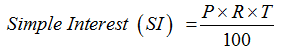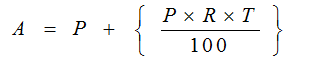In this lesson, we cover the absolute basics of Simple Interest. The purpose of this lesson is to help you answer one simple question: What is Simple Interest?

Definition of the term Interest: Interest is actually one of the most fundamental business terms, and without it, the financial trading of the world would come to standstill. Interest is defined as the “time value of money”.

What exactly does this mean?
Well, look at this way: with time, the value of money changes. Suppose you have Rs. 100 in the year 2000. Would it still be Rs. 100 or would the amount have grown? If you had deposited the money in a saving bank account, say with an annual rate of interest 4%, that money would have definitely grown by now. Can you calculate the amount you would have with you in 2013? Well, in case you can’t right now, go through these concept notes and you would know the answer.

The concept of simple and compound interest is especially applicable to the world of banking and economics. Whenever we borrow a certain sum of money (known as the principal), we pay back the original amount accompanied with a certain amount of interest on that amount. In a way, those are the charges of borrowing that sum of money. Simple interest is one method of determining the amount due at the end of loan duration. Another method of interest application is compound interest, but we study about it in next article.

Simple Interest Tooltip1: The Definitions
Principal (P): The original sum of money loaned/deposited. Also known as capital.
Interest (I): The amount of money that you pay to borrow money or the amount of money that you earn on a deposit.
Time (T): The duration for which the money is borrowed/deposited.
Rate of Interest (R): The percent of interest that you pay for money borrowed, or earn for money deposited

Simple Interest Tooltip 2: The FormulaWhere:
P: Principal (original amount)
R: Rate of Interest (in %)
T: Time period (yearly, half-yearly etc.)

Amount Due at the end of the time period, A = P (original amount) + SIIf you have a close look, Simple Interest is nothing else but an application of the concept of percentages.

Simple Interest Tooltip 3: Basic Problems to explain the concept

Basic Problem 1: What is the SI on Rs. 7500/- at the rate of 12% per annum for 8 years?

Using the Basic Formula:
Simple Interest (SI) = (P x R x T)/100
P – Principal amount, T- Number of years, R – Rate of Interest

Given P = 7500, T = 8 Years, R = 12%
Simple Interest (S.I.) = (7500X12X8)/100
Simple Interest (S.I.) = 7200

Basic Problem 2: A man borrowed Rs 15000/- at the rate of 24% SI and to clear the debt after 6years, much he has to return:

Using the Basic Formula:
Simple Interest (SI) = (P x R x T)/100
P – Principal amount, T- Number of years, R – Rate of Interest
Given P = 15000, T = 6 Years, R = 24%
Simple Interest (S.I.) = (15000X24X6)/100= Rs 21600
Therefore, total interest = 21600
Total repayment = S.I + Principal amount = 21600 + 15000 = Rs 36600

Basic Problem 3: A man borrowed Rs.12000 at the rate of 10% SI, and lent the same sum toanother person at the rate of 15% what will be the gain after 5 years?

Using the Basic Formula:
Simple Interest (S.I.) = (P x R x T)/100
P – Principal amount, T- Number of years, R – Rate of Interest

The man borrowed at 10% and he lent the same sum to another person at 15%
Therefore, his gain is actually equal to the different in the interest rate (per year)
= 15 – 10 =5% for 1 year
Thus, to calculate his gain, we use this difference as the rate of interest.
Given T = 5 years and P = Rs. 12000
Amount Gained = (12000x5x5)/100 = Rs 3000
Therefore, his gain = Rs 3000/-